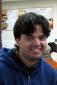# 'Linear Growth Curve Model, Path Specification' NPSOL returned a non-zero status code 1

3 posts / 0 newOffline
Joined: 09/16/2009 - 03:45
'Linear Growth Curve Model, Path Specification' NPSOL returned a non-zero status code 1

Hey folks,

Can someone give me a hand on what I'm doing wrong, please?

Thanks,
Carlos.

require(OpenMx)

# data

names(myLongitudinalData)

growthCurveModel <- mxModel("Linear Growth Curve Model, Path Specification",
type="RAM",
mxData(myLongitudinalData,
type="raw"),
manifestVars=c("members1", "members2", "members3"),
latentVars=c("intercept","slope"),
# residual variances
mxPath(from=c("members1", "members2", "members3"),
arrows=2,
free=TRUE,
values = c(0.8, 0.8, 0.8),
labels=c("residual","residual","residual")
),
# latent variances and covariance
mxPath(from=c("intercept","slope"),
arrows=2,
all=TRUE,
free=TRUE,
values=c(0.8, 0.5, 0.5, 0.8),
labels=c("vari", "cov", "cov", "vars")
),
mxPath(from="intercept",
to=c("members1", "members2", "members3"),
arrows=1,
free=FALSE,
values=c(1, 1, 1)
),
mxPath(from="slope",
to=c("members1", "members2", "members3"),
arrows=1,
free=FALSE,
values=c(0, 1, 2)),
# manifest means
mxPath(from="one",
to=c("members1", "members2", "members3"),
arrows=1,
free=TRUE,
values=c(0.2, 0.2, 0.2)),
# latent means
mxPath(from="one",
to=c("intercept", "slope"),
arrows=1,
free=TRUE,
values=c(0.2, 0.2),
labels=c("meani", "means")
)
) # close model
growthCurveFit <- mxRun(growthCurveModel)

Warning message:
In model 'Linear Growth Curve Model, Path Specification' NPSOL returned a non-zero status code 1. The final iterate satisfies the optimality conditions to the accuracy requested, but the sequence of iterates has not yet converged. NPSOL was terminated because no further improvement could be made in the merit function (Mx status GREEN).Offline
Joined: 07/31/2009 - 14:25
Code green means that nothing

Code green means that nothing was "wrong", but the code says the solution, while close enough, was still getting better with each iteration when the maximum iterations was reached (AFAIK).

You might want to play with starting values too ensure that the solution reached is stable.Offline
Joined: 07/30/2009 - 14:03
This warning is due to the

This warning is due to the solution space being "flat" near the optimum. That is to say, one solution is not that much better fitting than another.

One consequence of this is that confidence intervals on your parameters are likely to be large. Once the confidence interval search routine is implemented (soon!) you will be able to see just how "large" is "large".

In general, this may mean that the data you have is not doing a really great job of being fit by the model you've chosen. Something may be empirically underidentified. While code greens are routinely ignored by many people, I often take it as a hint that I have more work to do either in sampling data or thinking about how I might change my model.